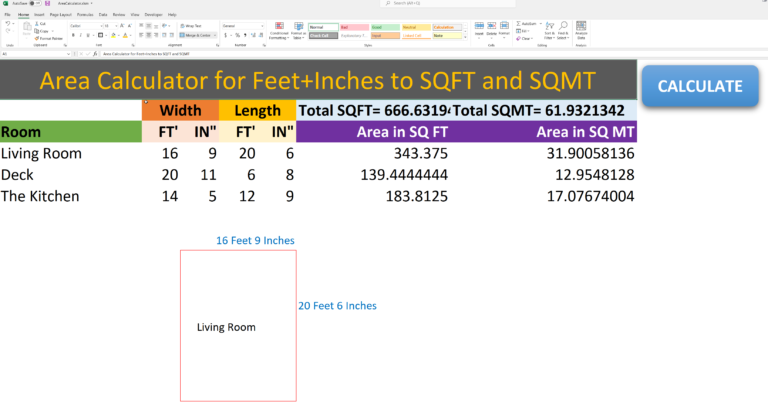# Area Feet and Inches Calculator in Excel

## Convert and calculate Feet and Inches into Square Feet

This excel calculator will calculate the area of any room in square feet or square meters, based on the length and width values entered in feet and inches.
You may enter only feet, only inches or a combination of both in either the width or length fields. The area of the room is automatically calculated and is displayed in square feet and square meters.You can also view the total area of the entire house or building in square feet and square meters.

Convert Feet Inches Area Calculator – Excel Template

## How To Calculate Square Footage

5 1 vote
Article Rating
Subscribe
Notify ofInline FeedbacksIt would be nice if could have shown with working of formulas used in your sheetDoesn’t show calculations and the downlowd doesn’t work without the macro.Do you have a formula table for linear feet also? This is great! Thank you

6
0